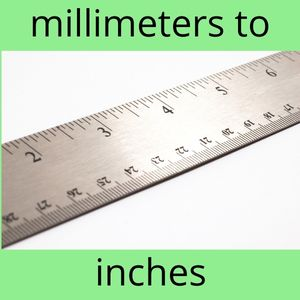## Millimeters to Inches Calculator

Enter Millimeters in the top textbox. Click Convert. Results appear below in Inches (rounded down).

Millimeters (mm)
Inches (in)## Millimeters to Inches List

30 millimeters to inches equals 1.
40 millimeters to inches equals 1.
50 millimeters to inches equals 1.
60 millimeters to inches equals 2.
70 millimeters to inches equals 2.
80 millimeters to inches equals 3.
90 millimeters to inches equals 3.
100 millimeters to inches equals 3.
200 millimeters to inches equals 7.
300 millimeters to inches equals 11.
400 millimeters to inches equals 15.
500 millimeters to inches equals 19.
600 millimeters to inches equals 23.
700 millimeters to inches equals 27.
800 millimeters to inches equals 31.
900 millimeters to inches equals 35.
1000 millimeters to inches equals 39.